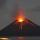# Cone + unit conversion - examples

1. CanopyMr Peter has metal roof cone shape with a height of 127 cm and radius 130 cm over well. He needs paint the roof with anticorrosion. How many kg of color must he buy if the manufacturer specifies the consumption of 1 kg to 3.3 m2?
2. Rotating cone IICalculate area of surface of rotating cone with base radius r=19 cm and height h=9 cm.
3. Ice cream in coneIn the ice cream cone with a diameter of 5.2 cm is 1.3 dl of ice cream. Calculate the depth of the cone.
4. Rotating coneCalculate the volume and the surface area of a rotating cone of base radius r = 2.3 dm and a height h = 46 mm.
5. VolcanoThe crater of a volcano is approximately in the shape of a cone of a base 3.1416 sq. Mi. The crater's depth is 1500 ft. How many cubic yards of earth would be required to fill this cavity?

We apologize, but in this category are not a lot of examples.
Do you have an interesting mathematical example that you can't solve it? Enter it, and we can try to solve it.

To this e-mail address, we will reply solution; solved examples are also published here. Please enter e-mail correctly and check whether you don't have a full mailbox.